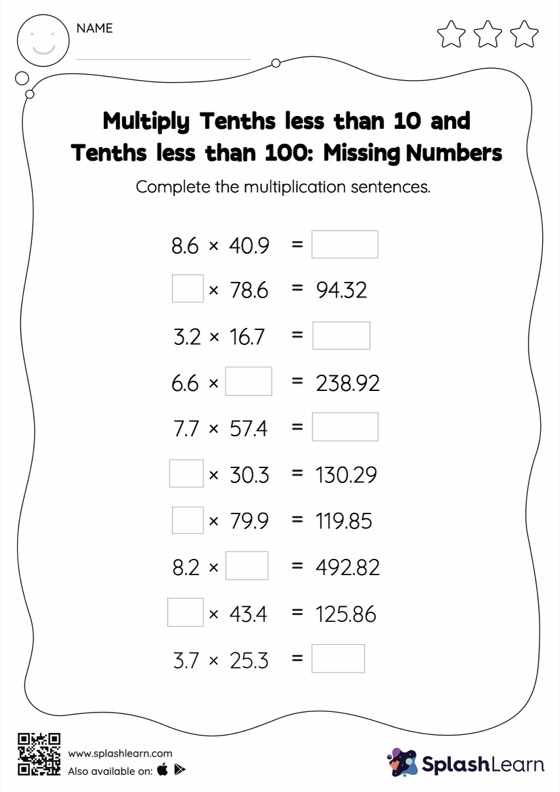# Multiply Tenths less than 10 and Tenths less than 100: Missing Numbers Worksheet

Home > Multiply Tenths less than 10 and Tenths less than 100: Missing NumbersThis worksheet invites students to multiply tenths less than 10 and tenths less than 100, which helps them become proficient in multiplication. The product of a tenth multiplied by another tenth will have two decimal digits. Multiply tenths less than 10 and tenths less than 100 worksheet is a great way to practice this concept as they find missing numbers in decimal multiplication.